International
Tables for
Crystallography
Volume H
Powder diffraction
Edited by C. J. Gilmore, J. A. Kaduk and H. Schenk

International Tables for Crystallography (2018). Vol. H, ch. 2.2, pp. 51-54

## Section 2.2.2. Production of synchrotron radiation

A. Fitcha*

aESRF, 71 Avenue des Martyrs, CS40220, 38043 Grenoble Cedex 9, France
Correspondence e-mail: fitch@esrf.fr

### 2.2.2. Production of synchrotron radiation

| top | pdf |

Synchrotron radiation is emitted by charged particles travelling at relativistic speeds when they are accelerated to move in a curved trajectory. In a modern synchrotron facility dedicated to the production of X-ray beams for scientific experiments, electrons are circulated in a closed horizontal orbit in a storage ring at an energy of several GeV, steered by magnetic fields from bending magnets. The overall circumference of the orbit can be several hundred metres depending on the design and specifications of an individual ring. The synchrotron ring is built up of cells (Fig. 2.2.1) comprising a straight section and a bending magnet by which the electrons are guided into the following straight section. Beamlines emerge tangentially from the bending magnets where synchrotron radiation is emitted by the electrons as they curve from one straight section into the next. Beamlines are also constructed on the straight sections where insertion devices, arrays of magnets providing an alternating magnetic field, are placed to cause the path of the electrons to oscillate and so also emit synchrotron radiation. By choosing the period of the magnetic array and by varying the strength of the magnetic field, the wavelength distribution and divergence of the X-rays emitted from an insertion device can be controlled. A straight section may accommodate more than one insertion device in series, allowing greater intensity or flexibility in the emitted radiation for the associated beamline. In the storage ring, the energy that the electrons lose by emitting synchrotron radiation is replaced by coupling the electrons to radio-frequency radiation supplied from klystrons or solid-state devices. Thus the synchrotron facility converts electrical energy, via radio waves and relativistic electrons, into powerful beams of electromagnetic radiation.Figure 2.2.1 | top | pdf |Schematic representation of a synchrotron storage ring with beamlines radiating tangentially from the bending magnets and in line with the straight sections. ID = insertion device, BM = bending magnet; RF = radio-frequency.

One key parameter of a storage ring is the energy of the circulating electrons. The energy of an electron moving with speed v is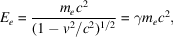where me is the rest mass of the electron, 9.10938356 (11) × 10−31 kg. The term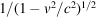is referred to as γ and is the factor by which the mass of the electron increases from its rest mass because of its relativistic speed. Expressed in eV (the conversion factor from kg to eV is c2/e), the electron rest mass is 5.109989461 (31) × 105 eV, so that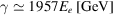when Ee is given in the customary units of GeV. Thus for a 3-GeV machine, a common energy for a synchrotron-radiation source, γ has the value of 5871. The mass of an electron with energy 3 GeV is therefore 3.22 atomic mass units, so around 7% more massive than a stationary atom of 3H or 3He.

Electrons do not circulate individually in the storage ring but in a series of bunches that are in phase with the accelerating radio frequency. Radiation is therefore emitted in pulses as each bunch passes through a bending magnet or insertion device. Thus the number and distribution of the electron bunches around the orbit determine the time structure of the emitted radiation. For most powder-diffraction applications using synchrotron radiation, the pulsed nature of the source can be neglected and the radiation can be regarded as continuous, although attention should also be paid to the performance of detectors that are more susceptible to pulse pile-up problems when the radiation arrives at very high average rates or in concentrated bursts (Cousins, 1994; Laundy & Collins, 2003; Honkimäki & Suortti, 2007), which can happen with certain bunch-filling modes. Certain specialized experiments requiring very fast time resolution can exploit the time structure of the source. In such experiments the longitudinal dimension of the bunches controls the pulse duration, which is usually a few tens of picoseconds.

In discussing the performance of different beamlines, the spectral brightness (Mills et al., 2005) is often quoted for the source and is defined as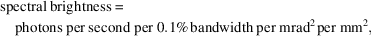where 0.1% bandwidth' represents δλ/λ = 0.001, the mrad2 term expresses the solid-angle of the emission of the X-rays from the source and the mm2 term relates to the cross-sectional area of the source. Thus a source of high spectral brightness emits many photons per second of the specified energy, into a narrow solid angle, with a small source size. The source size, which may well differ in the horizontal and vertical directions, is an important consideration as source size and beam divergence ultimately limit the performance of the beamline optical system in terms of collimation, energy resolution and focal spot size.

#### 2.2.2.1. Bending magnets

| top | pdf |

A bending magnet provides a vertical magnetic field to deflect the electrons laterally in the horizontal plane from a straight-line trajectory, and thereby causes the emission of synchrotron radiation (see Fig. 2.2.2). The lateral Lorentz force, F, acting on an electron travelling at velocity v in a magnetic field B is mutually perpendicular to both the magnetic field and the direction of travel of the electron, and is given by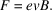Figure 2.2.2 | top | pdf |Emission of a fan of radiation by the electron beam as it curves in a bending magnet from one straight section of the ring to the next.

In a bending magnet the magnetic field is applied over an extended distance leading to a curved path of radius ρ. The centripetal acceleration is Fme, which for circular motion is equal to v2/ρ. Since vc,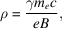so the radius of curvature decreases with magnetic field strength and increases with machine energy via increased γ. With the electron energy expressed in GeV, this can be approximated to ρ ≃ 3.34Ee [GeV]/B (where 109/c ≃ 3.34).

Synchrotron radiation is emitted in a forward cone tangential to the direction of the electrons' motion (Fig. 2.2.3) with a nominal Gaussian distribution and an opening angle of the order of 1/γ. Thus the radiation is highly collimated in the vertical plane. In the horizontal plane, synchrotron radiation is emitted in a broad fan, tangential to the curved trajectory of the electrons as they sweep through the bending magnet. Only a fraction of the radiation emitted by a bending magnet enters the associated beamline via a cooled aperture defining a horizontal acceptance angle of a few mrad. The radiation is polarized in the plane of the synchrotron orbit. Sometimes, more than one beamline can be built on a bending magnet with a suitable angular separation between them.Figure 2.2.3 | top | pdf |Synchrotron radiation is emitted in a cone of opening angle of the order of 1/γ tangential to the electrons as they follow a curved trajectory through the bending magnet.

Photons are emitted over a broad spectral range. The critical photon energy,c, divides the emitted power into equal halves and is given by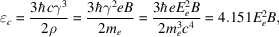or, with photon and electron energies in keV and GeV, respectively,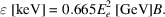The higher the critical energy, the greater the number of photons produced with short X-ray wavelengths. As an example, consider a bending magnet at the ESRF in Grenoble, France, which has a 6-GeV storage ring and bending magnets with a field of 0.85 T. The bending radius is 23.5 m and the critical photon energy is 20.3 keV (equivalent to a wavelength of 0.61 Å). The spectrum of such a device is shown in Fig. 2.2.4.Figure 2.2.4 | top | pdf |Spectrum of a bending magnet (B = 0.85 T) at the ESRF with an electron energy of 6 GeV (γ = 11 742), shown as flux per horizontal mrad for a 0.1% energy bandwidth at a storage-ring current of 200 mA. The critical energy of 20.3 keV divides the emitted power into equal halves.

The vertical collimation of the radiation varies with photon energy in a nonlinear manner (Kim, 2001). Nevertheless, the divergence decreases with increased photon energy, so beams with the shortest wavelengths are the most vertically collimated. Various approximations can be written to describe the variation, such as for a single electron (Margaritondo, 1988),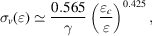where σv() is the standard deviation of the vertical-divergence distribution of photons of energy. For a population of electrons circulating in a storage ring, the distribution of the trajectories with respect to the plane of the orbit (of the order µrad) must also be considered, as these add to the vertical emission distribution. An approximation such as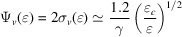will often be adequate to estimate the vertical divergence Ψv in the vicinity ofc. Thus for the bending magnet illustrated in Fig. 2.2.4, photons at the critical energy of 20.3 keV will have a vertical divergence of ∼100 µrad. A beamline would probably accept less than this, e.g. a 1.5-mm-high slit at 25 m from the source defining the beam onto a monochromator crystal defines an angle of ∼60 µrad.

#### 2.2.2.2. Insertion devices

| top | pdf |

Insertion devices can be classified into two main types, termed wigglers' and undulators', illustrated in Fig. 2.2.5. A wiggler has a relatively long magnetic period and the radiation from each oscillation is emitted like a series of powerful bending magnets, summing together to provide increased intensity. An undulator has a relatively short magnetic period and the radiation from sequential oscillations interferes coherently to give modified beam characteristics.Figure 2.2.5 | top | pdf |Schematic illustration of a wiggler (upper) and an undulator (lower).

For insertion devices the magnetic field acting on the electrons varies sinusoidally along the device,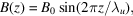where B0 is the peak magnetic field, z is the distance along the insertion-device axis and λu is the magnetic period. With a vertical field, the alternating magnetic field causes the electron path to oscillate in the horizontal plane. Note that the radiation is emitted mainly towards the outsides of the oscillations where the electrons change transverse direction, and where the magnetic field and beam-path curvature are highest. The maximum angular deflection of an electron from the axis of the insertion device is K/γ, where the deflection parameter K is given by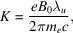which simplifies to K = 0.0934B0λu [mm] with λu expressed in mm. K is a crucial parameter that determines the behaviour of the insertion device.

#### 2.2.2.2.1. Wigglers

| top | pdf |

If K is large (10 or above), the insertion device is a wiggler and the electrons oscillate with an amplitude significantly greater than the emitted radiation's natural opening angle 1/γ. Every oscillation along the device produces a burst of synchrotron radiation and these add together incoherently so increasing the flux in proportion to the number of magnetic periods. The radiation emerges from the wiggler in a horizontal fan with a horizontal opening angle ∼2K/γ. The intensity of a wiggler-based beamline can be very high because each oscillation produces synchrotron radiation, and this radiation is directed close to the axis of the device. Like a bending magnet, wigglers produce a continuous spectrum but with the critical energy shifted to harder energies because the magnetic field is (usually) greater. Thus for a wiggler at a 6-GeV source, with a magnetic field of 1.2 T and a magnetic period of 125 mm, K is 14, the maximum deflection of the electrons from the straight-line path is 1.2 mrad and the critical photon energy is 28.7 keV. Magnetic fields of several tesla can be exploited using superconducting magnets to obtain even higher critical photon energies.

#### 2.2.2.2.2. Undulators

| top | pdf |

If the value of K is 2 or less, the insertion device is an undulator. The deflection of the electrons is comparable to the natural opening angle of the emitted radiation 1/γ. Radiation emitted from sequential oscillations interferes coherently, and the beam becomes highly collimated in the horizontal and vertical directions. Thus, the radiation from an undulator is concentrated into a central on-axis cone (fundamental and odd harmonics), surrounded by rings from higher-order even harmonics. The flux density arriving on a small sample from this central cone is therefore very high. With high on-axis intensity, it is therefore the undulators that provide the beams with the highest spectral brightness at any synchrotron-radiation source. The interference also modifies the spectrum of the device, which has a series of harmonics derived from a fundamental energy. At a horizontal angle θ to the axis of the insertion device, the wavelength of harmonic n is given by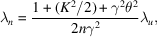which can be simplified on axis (θ = 0) to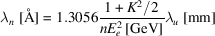or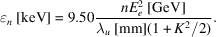On axis, only odd-numbered harmonics are emitted and it is these that are usually employed in a powder-diffraction experiment. The horizontal and vertical divergence of the radiation emerging from an undulator is of the order of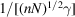, where N is the number of magnetic periods making up the device. The spectrum of an undulator at a 6-GeV source with a 35-mm magnetic period is shown in Fig. 2.2.6. By carefully shimming the magnetic lattice so that it is highly regular, the higher-order harmonics persist, allowing the undulator to be a powerful source of high-energy X-rays. Any imperfections in the magnetic periodicity cause the higher-order harmonics to broaden and fade away, reducing the utility of the device at higher energies.Figure 2.2.6 | top | pdf |Photon flux versus energy through a 1-mm2 aperture 30 m from the source, 0.1% bandwidth, for an ESRF u35 undulator (magnetic periodicity 35 mm, 1.6 m long, magnetic gap of 11 mm, peak magnetic field B0 = 0.71 T, electron energy 6 GeV, K = 2.31, storage-ring current 200 mA). Odd-numbered harmonics are labelled, which are those usually employed for powder-diffraction experiments as they have maximum intensity on axis.

#### 2.2.2.2.3. Tuning

| top | pdf |

For insertion devices the magnetic field can be modified by changing the vertical distance between the magnetic poles. By opening the gap, the magnetic field and K decrease following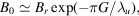where Br is proportional to the remanent magnetic field, which depends upon the nature of the magnets used in the insertion device, and G is the magnetic gap. Decreasing K for an undulator means that the energy of the fundamental harmonic increases; however, this is at the expense of the intensities of the higher harmonics. Thus the insertion device can be tuned to produce high intensity at the wavelength most suitable for a particular measurement. The smallest gap possible for a device depends on the design of the storage-ring vacuum vessel in which the electrons circulate. It is difficult to have a vessel smaller than about 10 mm high, and hence for an externally applied field a minimum magnetic gap of about 11 mm is to be expected. For smaller gaps, the magnets must be taken into the vacuum of the storage ring, a so-called in-vacuum' insertion device.

### References

Cousins, C. S. G. (1994). High-resolution diffraction at synchrotron sources: correction for counting losses. J. Appl. Cryst. 27, 159–163.Google Scholar
Honkimäki, V. & Suortti, P. (2007). Energy-dispersive diffraction with synchrotron radiation and a germanium detector. J. Synchrotron Rad. 14, 331–338.Google Scholar
Kim, K.-J. (2001). Characteristics of synchrotron radiation. X-ray Data Booklet, edited by A. C. Thompson & D. Vaughan. Lawrence Berkeley National Laboratory, USA. http://xdb.lbl.gov/Section2/Sec_2-1.html .Google Scholar
Laundy, D. & Collins, S. (2003). Counting statistics of X-ray detectors at high counting rates. J. Synchrotron Rad. 10, 214–218.Google Scholar
Margaritondo, G. (1988). Introduction to Synchrotron Radiation. Oxford University Press.Google Scholar
Mills, D. M., Helliwell, J. R., Kvick, Å., Ohta, T., Robinson, I. A. & Authier, A. (2005). Report of the Working Group on Synchrotron Radiation Nomenclature - brightness, spectral brightness or brilliance? J. Synchrotron Rad. 12, 385.Google Scholar